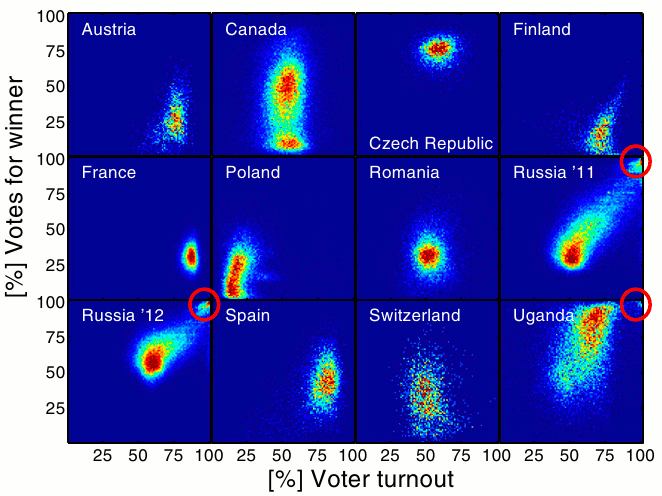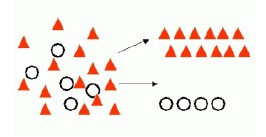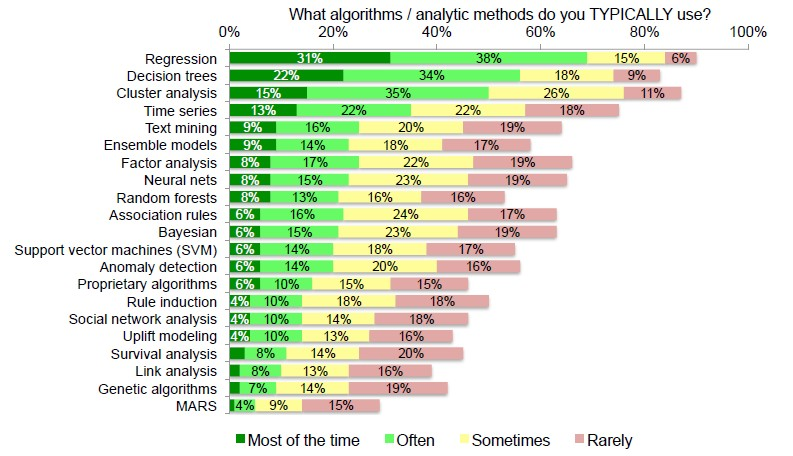# Data Mining - Support Vector Machines (SVM) algorithm

A support vector machine is a Classification method.

supervised algorithm used for:

Supports:

## Support vectors

The black line that separate the two cloud of class is right down the middle of a channel.

The separation is In 2d, a line, in 3D, a plane, in four or more dimensions an a hyperplane

Mathematically, the separation can be found by taking the two critical members, one for each class. This points are called support vectors.

These are the critical points (members) that define the channel.

The separation is then the perpendicular bisector of the line joining these two support vectors

That's the idea of support vector machine.

## Maximum margin hyperplane

Linear and Gaussian (non-linear) kernels are supported.

Distinct versions of SVM use different kernel functions to handle different types of data sets.

SVM regression tries to find a continuous function such that the maximum number of data points lie within an epsilon-wide tube around it.

SVM classification attempts to separate the target classes with this widest possible margin.

The maximum margin hyperplane is an other name for the boundary.

SVM can get more complex boundaries than a straight line thanks to the Kernel.

### Linear Kernel

Classes are called linearly separable if there exist a straight line that separates the two classes

In a straight line case, a simple equation gives the formula for the maximum margin hyperplane as a sum over the support vectors.

$$x = b + \sum_{i=1}^{\text{Nbr of Support Vectors}}{\alpha_i.y_i.a(i).a}$$

These are kind of a vector product with each of the support vectors, and the sum there.

It's pretty simple to calculate this maximum margin hyperplane once you've got the support vectors.

It's a very easy sum. It depends on the support vectors. None of the other points play any part in this calculation.

### Gaussian Kernel

In real life, you might not be able to drive a straight line between the classes

That makes support vector machines a little bit more complicated but it's still possible to define the maximum margin hyperplane under these conditions with Gaussian kernel.

By using different formulas for the kernel, you can get different shapes of boundaries, not just straight lines

SVMs excel at identifying complex boundaries but cost more computation time.

## Overfitting

Support vector machines are fantastic because they're very resilient to overfitting.

Support vector machines are naturally resistant to overfitting because any interior points aren't going to affect the boundary.

There's just a few of the points (2, 3, ..) in each cloud that define the position of the line: the support_vectors

All others instances in the training data could be deleted without changing the position of the dividing hyperplane

boundary depends only on a very few points so it's not going to overfit the dataset because it doesn't depend on almost all of the points in the dataset, just a few of these critical point – the support vectors

It's very resilient to overfitting, even with larges numbers of attributes

## Implementation

### Weka

• SMO: sequential minimum optimaztion. Works only with two classes data set. So use Multi-response linear regression or Pairwise linear regression
• LibSVm is an external library. LibSVM tools. Faster than SMO with more sophisticated options

## One class

One-class SVM builds a profile of one class and when applied, flags cases that are somehow different from that profile. This allows for the detection of rare cases that are not necessarily related to each other.

This is an anomaly detection algorithm which considers multiple attributes in various combinations to see what marks a record as anomalous.

It first finds the “normal” and then identifies how unlike this each record is – there is no sample set. The algorithm can use unstructured data, text, also and use nested transactional data (all the claims for a person for example).

This can be used to find anomalous records where you lack many examples.

Discover More(Machine learning|Inverse problems) - Regularization

Regularization refers to a process of introducing additional information in order to: solve an ill-posed problem or to prevent overfitting. This information is usually of the form of a penalty...Data Mining - (Anomaly|outlier) Detection

The goal of anomaly detection is to identify unusual or suspicious cases based on deviation from the norm within data that is seemingly homogeneous. Anomaly detection is an important tool: in data...Data Mining - (Classifier|Classification Function)

A classifier is a Supervised function (machine learning tool) where the learned (target) attribute is categorical (“nominal”) in order to classify. It is used after the learning process to classify...Data Mining - (Discriminative|conditional) models

Discriminative models, also called conditional models, are a class of models used in machine learning for modeling the dependence of an unobserved variable y on an observed variable x. Discriminative...Data Mining - Algorithms

An is a mathematical procedure for solving a specific kind of problem. For some data mining functions, you can choose among several algorithms. Algorithm Function Type Description Decision...Data Mining - Decision boundary Visualization

Classifiers create boundaries in instance space. Different classifiers have different biases. You can explore them by visualizing the classification boundaries. Logistic Regression method produces...Machine Learning - (Overfitting|Overtraining|Robust|Generalization) (Underfitting)

A learning algorithm is said to overfit if it is: more accurate in fitting known data (ie training data) (hindsight) but less accurate in predicting new data (ie test data) (foresight) Ie the model...ORE (Oracle R Enterprise)

Oracle R Enterprise is a component of Oracle...R

is a language and environment for statistical computing and graphics. Syntax is very similar to the S_(programming_language)S language. S is a language that was developed by John Chambers et al. at Bell...Statistics - Generalized Linear Models (GLM) - Extensions of the Linear Model

The Generalized Linear Model is an extension of the linear model that allows for lots of different,non-linear models to be tested in the context of regression. GLM is the mathematical framework used in...